# Algebra 1 : How to multiply integers

## Example Questions

1 2 3 4 6 Next →

### Example Question #51 : How To Multiply Integers

Multiply fifteen with eight.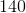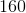Explanation:

Write the expression to express the question.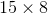Multiply the ones digits.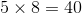Since this number has a tens digit, it will be used as a carryover.

Multiply the tens digits and add the carryover.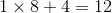Combine the numbers.

The answer is:### Example Question #52 : How To Multiply Integers

Multiply the following integers: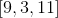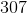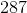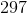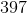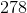Explanation:

The order of multiplication does not matter. We can choose to multiply eleven by three or nine to save work.

Multiply eleven with three.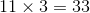Multiply thirty-three with nine.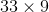Multiply the ones digits.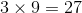The carryover is the tens place. Multiply the ones digit with the tens digit and add the carryover.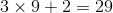Combine the numbers. The answer is.

### Example Question #53 : How To Multiply Integers

Multiply the numbers: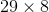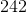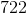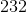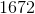Explanation:

Multiply the ones digits.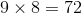The tens place will be the carryover for the next calculation.

Multiply the tens digit of 29 with the eight and add the carryover.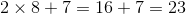Combine this number with the ones digit of the first calculation.

The answer is:### Example Question #54 : How To Multiply Integers

Multiply: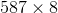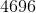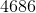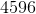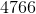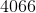Explanation:

Multiply the ones digit of 587 with the eight.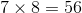The tens place is the carryover.

Multiply the tens digit of 587 with the eight and add the carryover.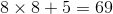The tens place is the carryover.

Multiply the hundreds digit of 587 with the eight and add the carryover.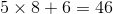Combine this number with the ones digits in the other calculations.

The answer is:### Example Question #55 : How To Multiply Integers

Multiply: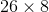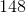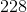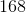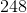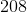Explanation:

Multiply the ones digit of 26 with eight.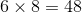The tens place is the carryover.

Multiply the tens digit of 26 with eight and add the carryover.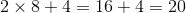Combine this number with the ones digit of the first calculation.

The answer is:### Example Question #56 : How To Multiply Integers

Solve: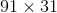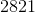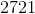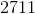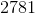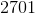Explanation:

Multiply 91 with the ones digit.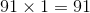Skip a line and multiply 91 with the tens digit.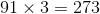Add a zero at the end of this number.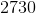Sum this number with the first number we have calculated.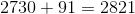The answer is:### Example Question #57 : How To Multiply Integers

Multiply the integers: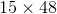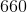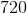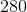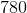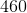Explanation:

Multiply the first number with the ones digit of the second number.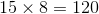Skip a line, and multiply the first number with the tens digit of the second number.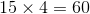We will need to add a zero to the right of this number.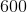Add this number with the first number.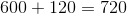The answer is:1 2 3 4 6 Next →